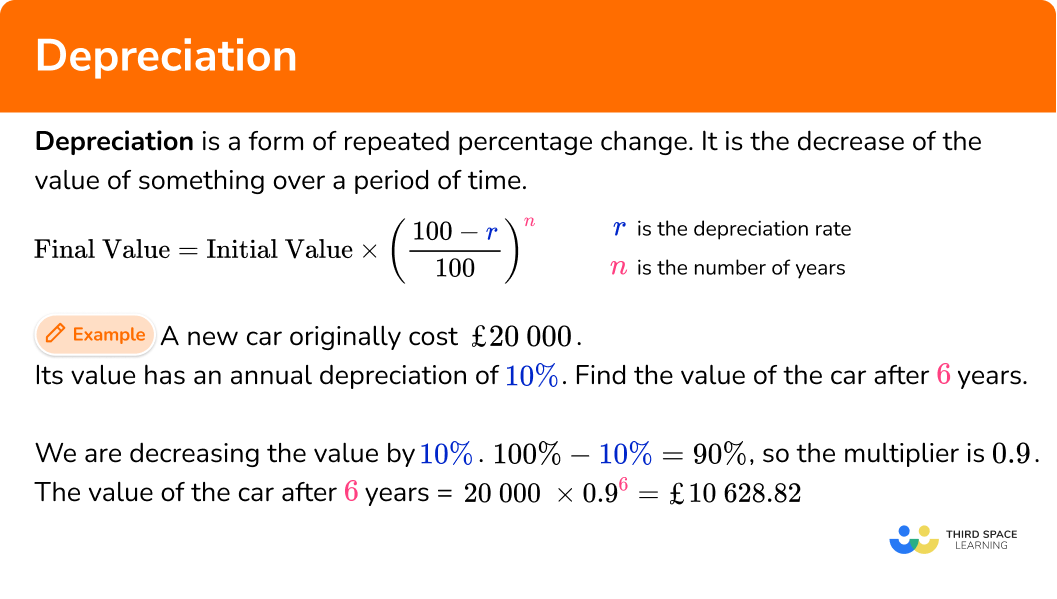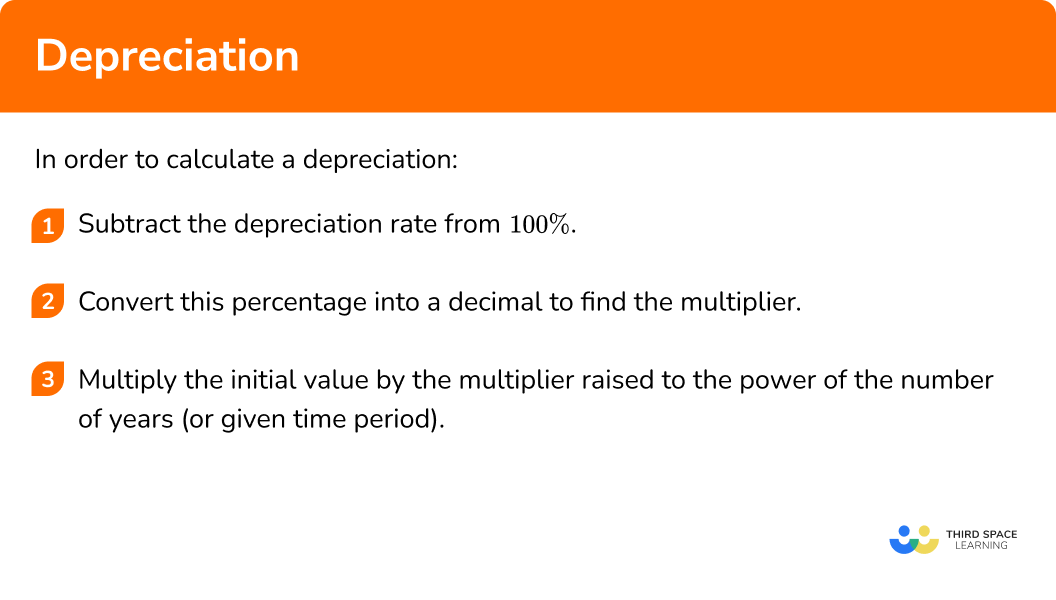# Depreciation

Here we will learn about depreciation, including what depreciation is and how to solve problems involving depreciation.

There are also depreciation worksheets based on Edexcel, AQA and OCR exam questions, along with further guidance on where to go next if you’re still stuck.

## What is depreciation?

Depreciation is a form of repeated percentage change. It is the decrease of the value of an asset over a period of time.

The rate of depreciation of an asset is usually given as a percentage and we can use this to find the percentage multiplier to reduce the cost of the asset each year over its lifespan.

Depreciation is calculated using the same method as calculating compound interest.

\text{Final Value} = \text{Initial Value} \times {{\left( \cfrac{100-r}{100} \right)}^{n}}

Where r is the percentage reduction and n is the number of years.

For example, a new car originally costs £20000. The car’s value has an annual depreciation of 10\%. Find the value of the car after 6 years.

The multiplier needed to reduce a value by 10\% will be 0.9 as we will be finding 90\% of the previous year’s value.

The value of the car after 6 years can be found by calculating,

20000 \times 0.9^{6}=10628.82.

So, after 6 years the car is worth £10628.82.

### What is depreciation?## How to calculate depreciation

In order to calculate a depreciation:

1. Subtract the depreciation rate from \bf{100\%} .
2. Convert this percentage into a decimal to find the multiplier.
3. Multiply the initial value by the multiplier raised to the power of the number of years (or given time period).

### Explain how to calculate depreciationDepreciation is part of our series of lessons to support revision on simple interest and compound interest. You may find it helpful to start with the main simple interest and compound interest lesson for a summary of what to expect, or use the step by step guides below for further detail on individual topics. Other lessons in this series include:

## Depreciation examples

### Example 1: finding a value after a depreciation

A boat is bought for £30000. It depreciates at a rate of 5\% per year. Find its value after 3 years.

1. Subtract the depreciation rate from \bf{100} .

100\%-5\% = 95\%

2Convert this percentage into a decimal to find the multiplier.

95\% = 0.95

3Multiply the initial value by the multiplier raised to the power of the number of years (or given time period).

30000 \times {{0.95}^{3}}=25721.25

After 3 years the boat is worth £25721.25.

### Example 2: finding a value after a depreciation with different rates

A car is bought for £50000. For the first 2 years it depreciates at a rate of 10\% per year. After that the depreciation rate changes to 6\%. Find its value after 7 years.

Subtract the depreciation rate from \bf{100} .

Convert this percentage into a decimal to find the multiplier.

Multiply the initial value by the multiplier raised to the power of the number of years (or given time period).

### Example 3: finding the number of years of depreciation

A motorbike is bought for £16000. It depreciates at a rate of 12\% per year. After n years the motorbike is worth £5754.15. Find n.

Subtract the depreciation rate from \bf{100} .

Convert this percentage into a decimal to find the multiplier.

Multiply the initial value by the multiplier raised to the power of the number of years (or given time period).

### Common misconceptions

• Using the depreciation rate without subtraction from \bf{100}

A common error is to think the multiplier for a 15\% depreciation rate is 0.15.

It is important to subtract the depreciation rate from 100 before finding the multiplier.

The multiplier for a 15\% depreciation is 0.85.

• Finding the reduction for \bf{1} year and then subtracting the same amount for the remaining years

A common error is to think that the amount the asset depreciates will remain constant each year. This is not the case. The depreciation rate may remain the same but as the value of the asset decreases so will the amount that is being subtracted.

If an asset is initially £100, and reduced by 10\%, \ £10 is subtracted to give £90.

If £90 is reduced by 10\%, \ £9 is subtracted to give £81.

### Practice depreciation questions

1. What multiplier should be used for a depreciation of 4\%?

1.040.960.040.94\begin{aligned} &100\%-4\% = 96\% \\\\ &96\%=0.96 \end{aligned}

2. What multiplier should be used for a depreciation of 27\%?

0.730.271.270.83\begin{aligned} &100\%-27\% = 73\% \\\\ &73\%=0.73 \end{aligned}

3. A car is bought for £12000 and depreciates at a rate of 8\% per year. Find its value after 3 years.

£6144£11040£9344.26£6.1412000 \times 0.92^{3}=9344.256

Rounded to 2 decimal places this is £9344.26.

4. A car is bought for £95000 and depreciates at a rate of 6.5\% per year. Find its value after 8 years.

£45600£88825£60419.63£55490.2395000\times {{0.935}^{8}}=55490.23

5. A boat is bought for £200000 and depreciates at a rate of 15\% per year for the first 3 years and then 9\% for the following 2 years. Find its value after 5 years.

£88741.06£124806.43£101711.38£108891.01200000\times {{0.85}^{3}}\times{{0.91}^{2}}=101711.38

6. A car is bought for £40000 and depreciates at a rate of 7\% per year. After n years it is worth £27827.53. Find n.

546740000\times {{0.93}^{5}}=27827.53

### Depreciation GCSE questions

1. What is the correct calculation to find the value of a car bought for £15000 after depreciating for 4 years at a rate of 2\% per year?

(1 mark)

15000\times {{0.98}^{4}}

(1)

2. A house is bought for £300000. For 2 years its value depreciates at a rate of 4\% per year.

For the further 3 years it depreciates at a rate of 1\% per year. What is the value of the house after 5 years?

(3 marks)

For a correct multiplier of 0.96 or 0.99 seen.

(1)

300000\times {{0.96}^{2}}\times{{0.99}^{3}} seen or calculating the amount after 2 years.

(1)

£268268.27

(1)

3. The value of a car depreciates at a rate of 13\% per year.

After how many years will the car be less than half its original value?

(3 marks)

Multiplier of 0.87 seen.

(1)

Attempts to apply powers to 0.87 of multiplying a value by powers of 0.87 .

(1)

5 years found.

(1)

## Learning checklist

You have now learned how to:

• Solve problems involving percentage change, including: percentage increase, decrease and original value problems and simple interest in financial mathematics

## Did you know?

Depreciation is important in business. A company must be aware of its market value (book value), know what assets are tied into the business, and know exactly what is happening with its cash flow.

A balance statement (balance sheet) is a financial document that shows the value of a company or organisation.

An income statement is a financial document that shows the income and expenditure of a company.

A cash flow statement provides information on all of the different ways cash is coming in and going out of the business including business activities and investments.

Assets are very important to a business. An asset is a useful or valuable item or person. Three different types of asset include:

• Tangible assets have a monetary value and usually a physical form (such as office equipment, a building, or an employee).
• Intangible assets have no monetary value or physical form (such as goodwill, a patent or trademark, or intellectual property).
• Fixed assets are long term tangible assets that make money however this type of asset is not expected to be used up or sold within its first year.

Assets within a business can be tax-deductible meaning that the tax is returned to the business. For tax purposes, a company should declare any assets that are tax deductible.

The useful life of the asset is the estimated number of years an asset is likely to remain in service. An asset’s useful life can be extended with the correct maintenance.

For example, vehicles have an annual service to extend their working life. The cost of a vehicle includes every debit needed to buy and maintain the vehicle: the purchase price (the original cost), maintenance, fuel, insurance, and tax.

Most tangible assets (for example, a piece of equipment) depreciate in monetary value over their lifetime. When the asset does not make money, the asset is a write off. A fixed asset depreciation schedule predicts the useful life of an asset. The residual value (salvage value) of the asset is an estimated value of an asset when it is no longer profitable to the business.

The total depreciation rate is an annual percentage of the total amount of depreciation for that year. The total amount of depreciation of a company’s assets is known as accumulated depreciation. Loan amortisation allows for a business to borrow money when they face a large cost. Repayments are spread over months to years. This allows small businesses to buy assets that can help to make the business more profitable in the future.

Depreciation calculators help determine the life of an asset. These include:

• The straight-line depreciation method
• The double-declining balance method
• The sum-of-the-years-digits (syd)

Each of these methods have their own advantages and disadvantages in helping businesses determine their future success. For example, accelerated depreciation methods including the sum of the years digits recognise higher depreciation expenses during the earlier years of a business starting up.

## Still stuck?

Prepare your KS4 students for maths GCSEs success with Third Space Learning. Weekly online one to one GCSE maths revision lessons delivered by expert maths tutors.

Find out more about our GCSE maths tuition programme.